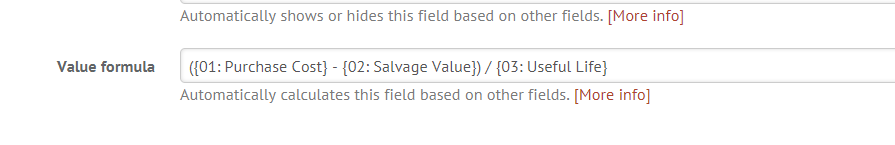Want to be able to track depreciation of an asset over its lifespan or have a way to automatically determine priority level for work requests? These options and MORE are now available with our Formula Custom Fields feature. For more information on this, see some of the examples below, or contact your Customer Success Manager today!

## Most commonly used formulas:

• Math Operators – Division and Multiplication
• Math Operators -- Subtraction and Addition
• Text Function - Includes
• && (And): Determines if both values are true.
• Logical Operators -  ? (Ternary)
• Text Function - Contains
• Text Operator - Concatenation
• Logical Operators - Or

## Example #1: Math Operators - Division and Multiplication

### Division: On the request:### Multiplication: Formula:To build this formula, you will need two number fields and another field to build out the equation (product field). From here you can build out the general math equation using the divide function, dividing the two number fields, and then the multiplication function, multiplying the total by 100 to give you the percentage.

## Example #2: Math Operators - Addition and Subtraction

### Formula:## Example #3: Text Function  - Includes

This operator determines if an array includes the given value.

### Formula:

This formula allows you to flag certain words or characters to trigger another field. In this example, we tagged the “5K” and “Fun Run” options as needing race setup. To build this formula, you will want to use the “Includes” function and then add in the options from the previous field that would like to include.

## Example #4: && (And): Determines if both values are true.

This formula will auto generate a response in one field if two other fields have been selected. If you want “x” & “y” to equal “z” and populate a new field.

Note - The following example is a formula combination of && as well as || (or). There is an example of this function at the end. If you just want one option then you would need the && symbol between the two fields you want to determine for the 3rd field.

## Example #5: Logical Operators -  ? (Ternary)

Returns the value of the first expression if the condition is true otherwise returns the value of the second. Aka: it will auto-populate one field if a particular value was selected in another.

## Example #6: Text Function - Contains

If a request has a long text field, and you want one field to flag key words such as “allergies” or “set up” then this is what you would want to use

## Example #7: Text Operator - Concatenation

If you have a customer that wants to have one field where they want several pieces of information to appear such as “maintenance notes” or “catering notes” then this would allow their users to have that a quicker glance than going through every field of a request

### Formula:

####Note - this formula looks kind of funky, but it is so that there is a line break between the fields that are being combined. Otherwise, this might be kind of confusing for those who are viewing it.

## Example #8: Logical Operators - Or (||)

This will be if one or the other is true, then it will populate that in the field. This can typically be combined with other functions that are listed above (such as And && or Ternary ?). It allows you to have multiple options for determining fields.

## Example #9: Math Operators - Straight Line Depreciation

The simplest method of calculating depreciation is to take the net asset value and divide it by the number of periods (usually years) of useful life. This can be done by using the Math Operators.

### Formula: